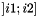# util::PrefixSumArray1DOfTInterval< T > Class Template Reference

skeleton of prefix sum array using the interval notation for 1d partitioning algorithm (this class does not actually exist). More...

`#include <util/template.hpp>`

## Detailed Description

### template<typename T> class util::PrefixSumArray1DOfTInterval< T >

skeleton of prefix sum array using the interval notation for 1d partitioning algorithm (this class does not actually exist).

Any type that provides the following functions can be used safely with 1D partitioning algorithms that use the interval notation. This class does not actually exist, it is provided for documentation purpose only. Notice that since the types are given as template parameter to the partitioning algorithm, there is no need to inherit from this class.

The interval notation differs from the bracket notation since in the in the bracket notation the load of an interval is equal to the sum of the load of its parts. This might not be true in the interval notation.

Notice that the class does not need to provide its size since it is given as a parameter to the 1D partitioning functions.

Types that respect this prototype include: util::Aggreg2Dto1Dpart, util::AggregMax2Dto1D and util::Aggreg2Dto1D.

Notice that a type that respect the bracket notation can easily be extended to respect the interval notation. However, the algorithm that runs on the interval notation are typically slower than their bracket notation counterpart.

List of all members.

## Public Member Functions

const Tinterval (int i1, int i2) const
returns the load of interval.

## Member Function Documentation

template<typename T >
 const T& util::PrefixSumArray1DOfTInterval< T >::interval ( int i1, int i2 ) const

returns the load of interval.

i1 and i2 must be positive (or null) and i2 must be less or equal toif the size of the original array is. In that sense, the interval notation is a "Pascal-style" array. The first element of the load is given by interval(0,1);

There is no constraint that i2 must be larger than i1. For instance, interval(1,0) is a valid function call that designate the load of the empty interval, that is to say, that returns 0. i1 can also be larger than the size of the array and designate the empty interval as well.

The only contraints on the function is that empty interval have a load of 0 and the load must be increasing by inclusion; that is to say if interval ]i1; i2] includes interval ]i3;i4] then interval(i1,i2)>=interval(i3,i4).

In particular, if i1<i2<i3, interval(i1,i3) might be different from interval(i1,i2)+interval(i2,i3); it might be less, it might be more, it might be the same.

The documentation for this class was generated from the following file: